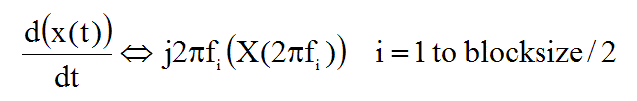# Tools | Differentiate

Performs differentiation on all (or selected) M#s in a Data Block.

All differentiation is done in the frequency domain.
Time domain waveforms are transformed to the frequency domain, differentiated, and transformed back to the time domain.

Frequency domain measurements are differentiated using the following equivalent operation,where:

x(t) = continuous time waveform.

Xi(2pf i) = linear spectrum (FFT ) of the signal for the ith sample.

2pf i = frequency of the ith sample (radians/second).

f i = frequency of the ith sample (Hz).

j - denotes the imaginary operator.

Time domain differentiation of a signal is equivalent to multiplying each sample of its frequency spectrum Xi(2pf i) by its sampled frequency 2pf i.